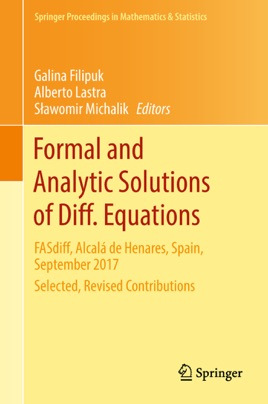• 119,99 €

## Description de l’éditeur

These proceedings provide methods, techniques, different mathematical tools and recent results in the study of formal and analytic solutions to Diff. (differential, partial differential, difference, q-difference, q-difference-differential.... ) Equations.  They consist of selected contributions from the conference "Formal and Analytic Solutions of Diff.  Equations", held at Alcalá de Henares, Spain during September 4-8, 2017. Their topics include summability and asymptotic study of both ordinary and partial differential equations. The volume is divided into four parts. The first paper is a survey of the elements of nonlinear analysis. It describes the algorithms to obtain asymptotic expansion of solutions of nonlinear algebraic, ordinary differential, partial differential equations, and of systems of such equations. Five works on formal and analytic solutions of PDEs are followed by five papers on the study of solutions of ODEs. The proceedings conclude with five works on related topics, generalizations and applications. All contributions have been peer reviewed by anonymous referees chosen among the experts on the subject.

The volume will be of interest to graduate students and researchers in theoretical and applied mathematics, physics and engineering seeking an overview of the recent trends in the theory of formal and analytic solutions of functional (differential, partial differential, difference, q-difference, q-difference-differential) equations in the complex domain.